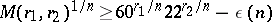# Odlyzko bounds

Effective lower bounds for $M ( r _ { 1 } , r _ { 2 } )$, the minimal value of the discriminant $| d ( K ) |$ of algebraic number fields $K$ having signature $( r _ { 1 } , r _ { 2 } )$ (i.e. having $r_1$ real and $2r_2$ non-real conjugates), obtained in 1976 by A.M. Odlyzko. See also Algebraic number; Number field.

The first such bound was proved in 1891 by H. Minkowski [a4], who showed

\begin{equation} \tag{a1} M ( r _ { 1 } , r _ { 2 } ) > \left( \frac { \pi } { 4 } \right) ^ { 2 r _ { 2 } } \left( \frac { n ^ { n } } { n ! } \right) ^ { 2 }, \end{equation}

with $n = r _ { 1 } + 2 r _ { 2 }$. He obtained it using methods from the geometry of numbers; the same method was used later by several authors to improve (a1) (see [a5] for the strongest result obtained in this way).

In 1974, H.M. Stark ([a11], [a12]) observed that Hadamard factorization of the Dedekind zeta-function $\zeta _ { K } ( s )$ leads to a formula expressing $\operatorname{log} | d ( K ) |$ by the zeros of $\zeta _ { K } ( s )$ and the value of its logarithmic derivative at a complex number $s _ { 0 } \neq 0,1$ with $\zeta_{ K } ( s _ { 0 } ) \neq 0$. He used this formula with a proper choice of $s_0$ to deduce lower bounds for $M ( r _ { 1 } , r _ { 2 } )$ which were essentially stronger than Minkowski's bound, but did not reach the bounds obtained by geometrical methods.

In 1976, Odlyzko [a7] (cf. [a9]) modified Stark's formula and obtained the following important improvement of (a1):(a2)

with $\operatorname { lim } _ { x \rightarrow \infty } \epsilon ( n ) = 0$.

In particular, one has

\begin{equation*} D = \liminf _ { n \rightarrow \infty } M ( r _ { 1 } , r _ { 2 } ) ^ { 1 / n } \geq 22. \end{equation*}

If the extended Riemann hypothesis is assumed (cf. also Riemann hypotheses; Zeta-function), then the constants $60$ and $22$ in (a2) can be replaced by $180$ and $41$, respectively. For small degrees the bound (a2) can be improved (see [a2], [a10]) and several exact values of $M ( r _ { 1 } , r _ { 2 } )$ are known.

On the other hand, it has been shown in [a13], as a consequence of their solution of the class field tower problem (cf. also Tower of fields; Class field theory), that $D$ is finite. The best explicit upper bound for it, $D \leq 92.4$, is due to J. Martinet [a1], who obtained this as a corollary of his constructions of infinite $2$-class towers of suitable fields.

For surveys of this topic, see [a9], [a3] and [a8].

How to Cite This Entry:
Odlyzko bounds. Encyclopedia of Mathematics. URL: http://encyclopediaofmath.org/index.php?title=Odlyzko_bounds&oldid=50738
This article was adapted from an original article by WÅ‚adysÅ‚aw Narkiewicz (originator), which appeared in Encyclopedia of Mathematics - ISBN 1402006098. See original article